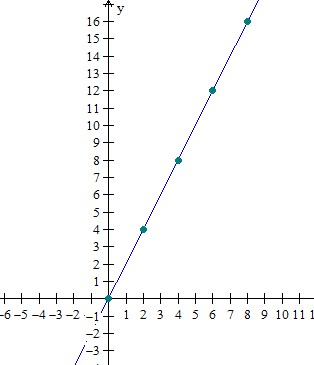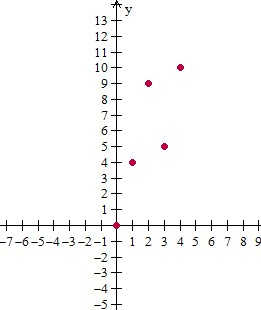# Graphing whole number functions

#### Excel Pivot Tables and Dashboard. Simple & Quick tutorial!

Most Popular

7 Lectures 58 mins

#### Learn HTML Tables

15 Lectures 59 mins

#### Excel Analytics - Data Analysis with Pivot-Tables and Charts

46 Lectures 2.5 hours

A function is a rule that takes in and works on an input and gives an output. It is usually represented as f(x) = y, where x is the input variable and y is the output variable.

By giving different whole number values to x, values of y can be found from the function rule. The x and y values are put in a table and then plotted as a graph.

Plotting a graph

So, from a function rule, x and y values can found and put in a table and also plotted as a graph.

Conversely, from a graph we can find x and y values and make a table of those values and find the function rule for the given graph also.

The table below describes a function. Graph this function.

Input(x) Output(y)
0 0
2 4
4 8
6 12
8 16

### Solution

Step 1:

From the table, we get the coordinates of the points as (0, 0), (2, 4), (4, 8), (6, 12), and (8, 16)

Step 2:

Making a plot of these points, we getThe graph of a function is given below. Fill in the table for this function.### Solution

Step 1:

From the graph plot, we get the coordinates of the points as (0, 0), (1, 4), (2, 9), (3, 5), and (4, 10)

Step 2:

Making a table of the x and y values, we get

Input(x) Output(y)
0 0
1 4
2 9
3 5
4 10